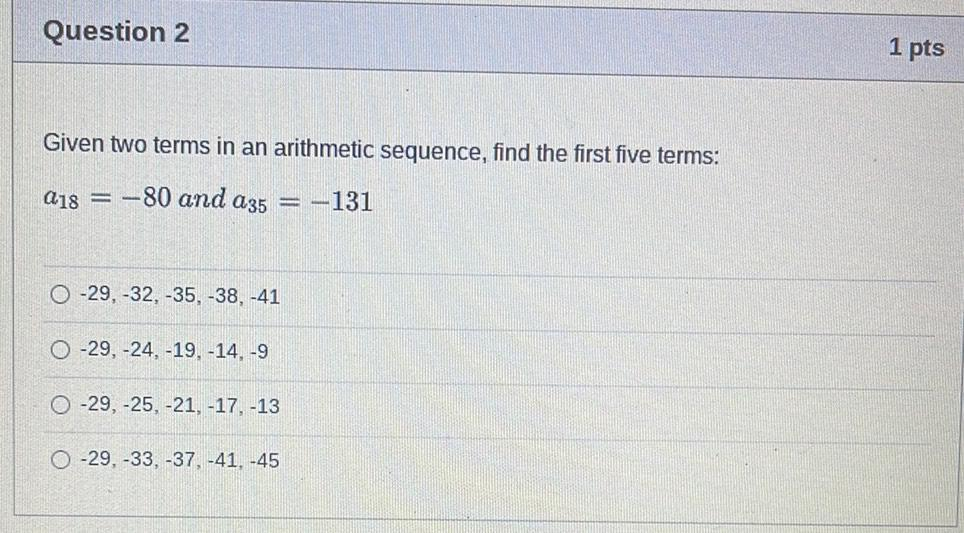Question:

# Question 2 1 pts Given two terms in an arithmetic sequence, find the first five terms: a18 = -80 and a35 = -131 O-29, -32, -35,Question 2 1 pts Given two terms in an arithmetic sequence, find the first five terms: a18 = -80 and a35 = -131 O-29, -32, -35, -38, -41 O-29, -24, -19.-14, -9 O -29, -25, -21, -17, -13 O -29, -33, -37, -41. -45